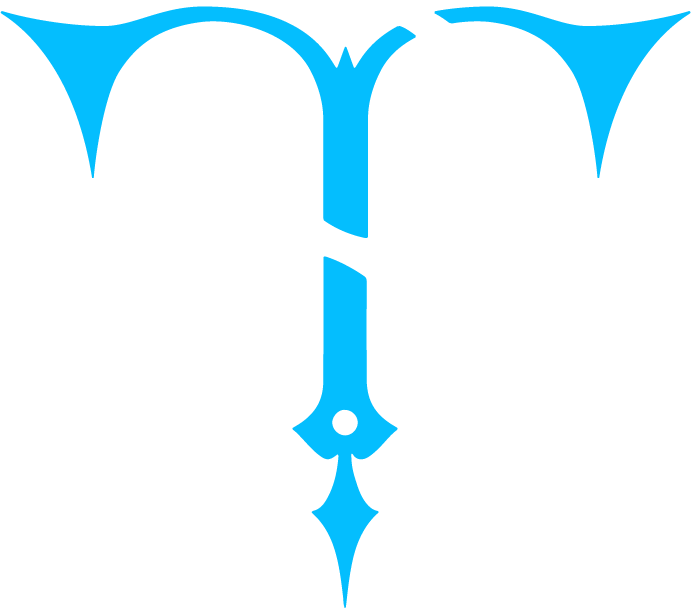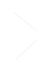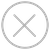TensorSpace.js
Getting StartBasic ConceptsTensorSpace ConverterModel PreprocessingModelsLayersMerge FunctionLayer Configuration
We can add configuration when creating TensorSpace Layer. There are two types of parameters. The first type of parameters are network related parameters, this kind of parameters are related to neural network structure, such as kernelSize, filters, strides for Conv2d, units for Dense, and so on. The second type of parameters are visualization related parameters, this kind of parameters are related to 3D visualization, such as initStatus, animeTime, and so on.
In different circumstances, configure TensorSpace Layer in different ways:
Init with a pre-trained model
If TensorSpace model init with a pre-trained model, for example, load a preprocessed TensorFlow model, we just need to configure some optional visualization related parameters for TensorSpace Layer. There is no need to configure network related parameters, as TensorSpace Model will automatically inject layer metrics based on pre-trained model in initialization process.
For example, to configure LeNet Layers with a pre-trained model:
``````let model = new TSP.models.Sequential( container );
initStatus: "open"
}) );
outputs: ["0", "1", "2", "3", "4", "5", "6", "7", "8", "9"]
}) );
type: "tensorflow",
url: "model.json"
});
model.init();``````
wb_sunnyNote:
If the pre-trained model has dynamical shape, it is required to configure the input shape in TensorSpace Input Layer. For example, the pre-trained InceptionV3 model from Keras Application has a dynamically shape, which make it has ability to accept input shape from [299, 299, 3] to [75, 75, 3]. If you would like to visualize this kinds of models in TensorSpace, it is required to configure a specific input shape for TensorSpace Input Layer. The input shape configuration would be like:
``````model.add( new TSP.layers.RGBInput({
shape: [299, 299, 3]
}) );``````
Init without a pre-trained model
If TensorSpace model init without a pre-trained model, which will be an empty TensorSpace model, we need to configure required network related parameters and some optional visualization parameters.
For example, to configure LeNet Layers without a pre-trained model:
``````let model = new TSP.models.Sequential( container );
shape: [28, 28, 1]
}) );
}) );
kernelSize: 5,
filters: 6,
strides: 1,
initStatus: "open"
}) );
poolSize: [2, 2],
strides: [2, 2]
}) );
kernelSize: 5,
filters: 16,
strides: 1
}) );
poolSize: [2, 2],
strides: [2, 2]
}) );